# Arduino

 In:=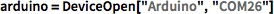X arduino = DeviceOpen["Arduino", "COM26"]
 Out=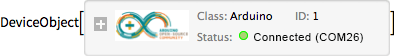In:=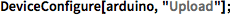X DeviceConfigure[arduino, "Upload"]
 In:=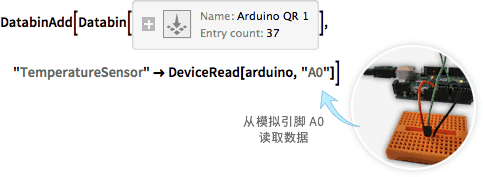X DatabinAdd[Databin["2anGrs92"], "TemperatureSensor" -> DeviceRead[arduino, "A0"]]

 In:=X arduino = DeviceOpen["Arduino", "COM26"]
 Out=In:=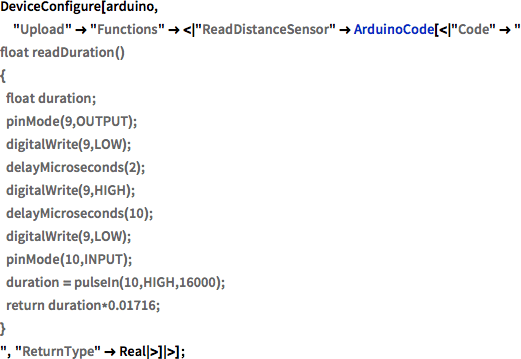X DeviceConfigure[arduino, "Upload" -> "Functions" -> <|"ReadDistanceSensor" -> ArduinoCode[<|"Code" -> " float readDuration() { float duration; pinMode(9,OUTPUT); digitalWrite(9,LOW); delayMicroseconds(2); digitalWrite(9,HIGH); delayMicroseconds(10); digitalWrite(9,LOW); pinMode(10,INPUT); duration = pulseIn(10,HIGH,16000); return duration*0.01716; } ", "ReturnType" -> Real |>] |>]

 In:=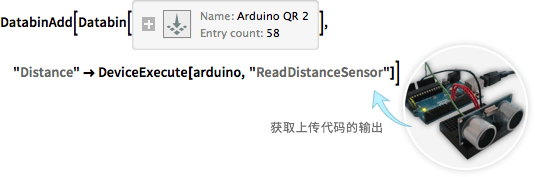X DatabinAdd[Databin["2anGrs92"], "Distance" -> DeviceExecute[arduino, "ReadDistanceSensor"]]